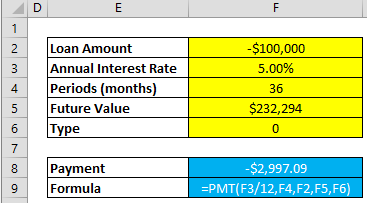Excel is an amazing tool for financial tasks, and calculating loan payments is no exception. The PMT function (sounds a lot like payment, right?) is often essential to investment, valuation, and decision making processes. If you are dealing with capital loans like mortgages, you’re going to want to waste no time to add it to your repertoire.

In this article, we cover the details of this essential function and how to use it to calculate loan payments. You can download our sample workbook below.## Syntax

PMT(rate, nper, pv, [fv], [type])

• rate: The interest rate for the loan
• nper: The total number of payments
• pv: The present value, or the total amount that a series of future payments is worth now – also known as the principal
• [fv]: Optional. The future value, or a cash balance you want to attain after the last payment. If fv is omitted, it is assumed to be 0 (zero), meaning that the future value of a loan is 0
• [type]: Optional. Available inputs 0 (zero) or 1 indicate when payments are due (at the beginning or end of each period)

## Examples

Let’s take a look at how this formula works on two examples. The PMT function calculates the repayment amount of a loan. One thing to note here is that the function assumes that payments and interest rate are always constant, which rarely is the case in real life.

The first scenario consists of a basic use case, where we work with the basic elements of a loan calculation and should work for most everyday calculations. The second case cover the use of optional parameters and is a bit more geared for specific situations.

## Scenario 1

Generally speaking, loan payments can be defined by three parameters,

1. Interest rate
2. Number of periods
3. Total loan amount

Using these as input, the PMT function calculates constant payment amount needed through the loan period. In this case, it is assumed that payments are paid at the end of each period, and the loan is to be paid in full.## Scenario 2

Let’s now take a closer look at the formula and try to use its full potential. Adding the two optional parameters, you can specify a target cash balance for after the last payment is made, and whether payments are to be made at the beginning of each period or the end.

This scenario can be considered an extended version of the first, we use the same first three arguments, but also add the two optional parameters of future value and payment type into the equation.## Tips

• If Loan Amount is entered as a positive number, the calculated payment amount will be negative. This is because the money is coming out of pocket. If you’d like to show negative numbers instead, just multiply everything with ‘-1’.
• The loan payments returned by the function includes principal and interest, but of course does not include any taxes, reserve payments, fees etc. one might want to consider.
• Rate and nper units should be consistent. If nper is defined in months, subsequently an annual interest rate should be divided by 12.
• Multiplying payment value by nper returns total amount paid.

Want to learn more about using Excel for finance? See our 10 most useful formulas in financial modeling.